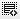## dataframe from series0

Hi,

I am trying to create a simple dataframe with two columns butits created as rows and when i add "columns" parameter the values are NAN

``````import pandas as pd
import numpy as np
import matplotlib.pyplot as plt
%matplotlib inline

col1 = pd.Series(np.arange(1,11))
print(col1)
col2

##shows col1 and col2 as rows
df = pd.DataFrame([col1,col2])
df

#shows col1 and col2 as columns but data is NAN
df = pd.DataFrame([col1,col2], columns=['a','b'])
df``````

Thanks!1

Hi,

If you use this form of DataFrame creation, col1 and col2 are the respective rows and not columns. Ideal method to achieve what you are looking for is:

``````df = pd.DataFrame({
'a': col1,
'b': col2
})``````

Alternatively, a not so appropriate method would be:

``df = pd.DataFrame([col1, col2], index=['a', 'b']).T``

Hope this solves the query.0

Perfect. Thank you so much !!

Click on thisicon to add code snippet.